# 模拟均匀磁场中的硅量子点

2021年 1月 26日

### 硅量子点的薛定谔方程

(1)

H=\frac{P_x^2}{2 m_\perp}
+\frac{P_y^2}{2 m_\perp}
+\frac{P_z^2}{2 m_\parallel}
+V(\mathbf{r})
+\mu_B \mathbf{B} \cdot \sigma

(2)

\mathbf{P}=i \hbar \nabla + e \mathbf{A}(\mathbf{r})

(3)

V(\mathbf{r})=\frac{1}{2} m_\perp \omega_x^2 x^2
+\frac{1}{2} m_\perp \omega_y^2 y^2
+q F_z z
+U_0 \Theta(z)

### 参数和变量

mxy 0.19 * me_const 1.7308E-31 kg 横向有效质量
mz 0.98 * me_const 8.9272E-31 kg 垂直有效质量
wx 1[meV]/hbar_const 1.5193E12 rad/s x 方向上的捕获势函数的角频率
wy 3*wx 4.5578E12 rad/s y 方向上的捕获势函数的角频率
Fz 10[MV/m] 1E7 V/m 电场
U0 3 [eV] 4.8065E-19 J 氧化物势垒
B e_const * hbar_const/2/me_const 9.274E-24 m2·A 玻尔磁子
B 1[T] 1T 磁通密度

Ax z*B/2 Wb/m 矢量势
Ay 0[Wb/m] Wb/m 矢量势
Az -x*B/2 Wb/m 矢量势

### 物理场设置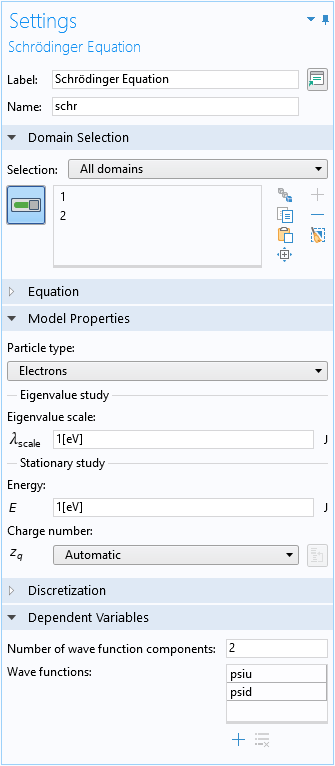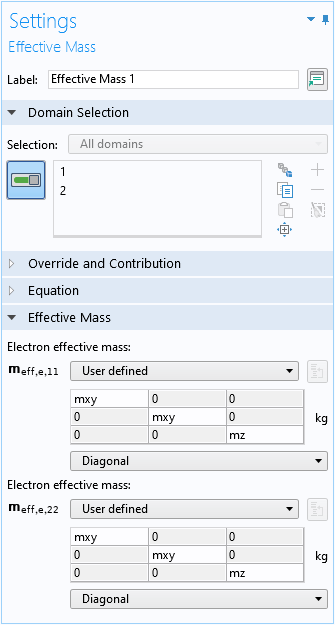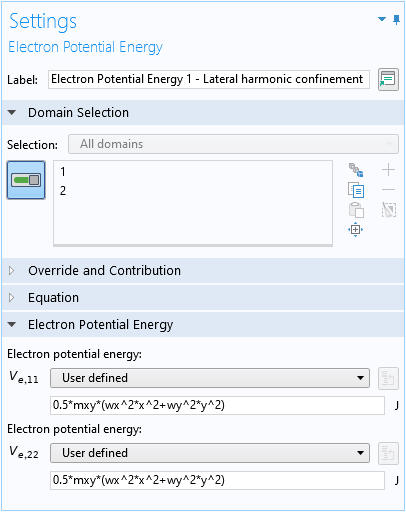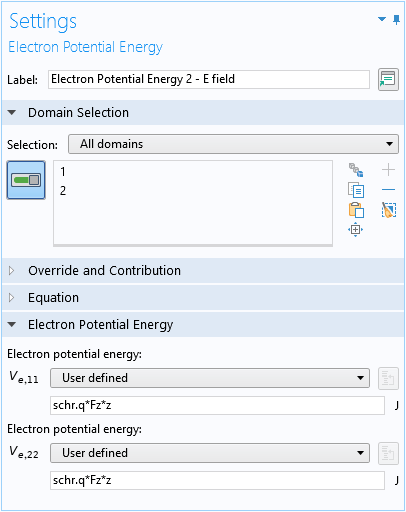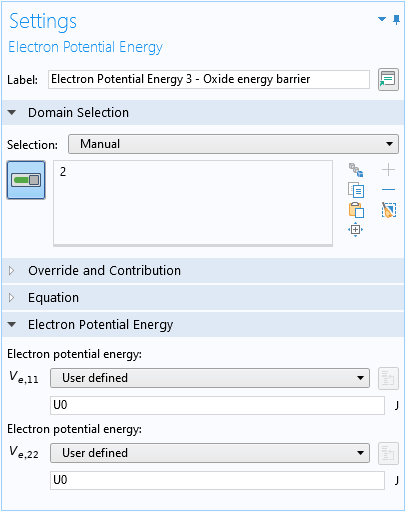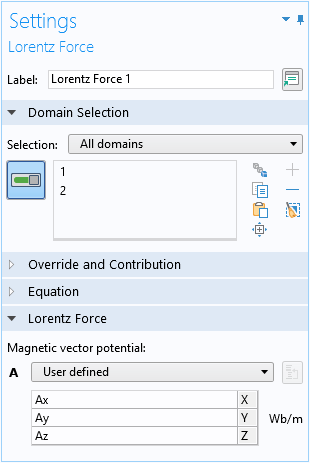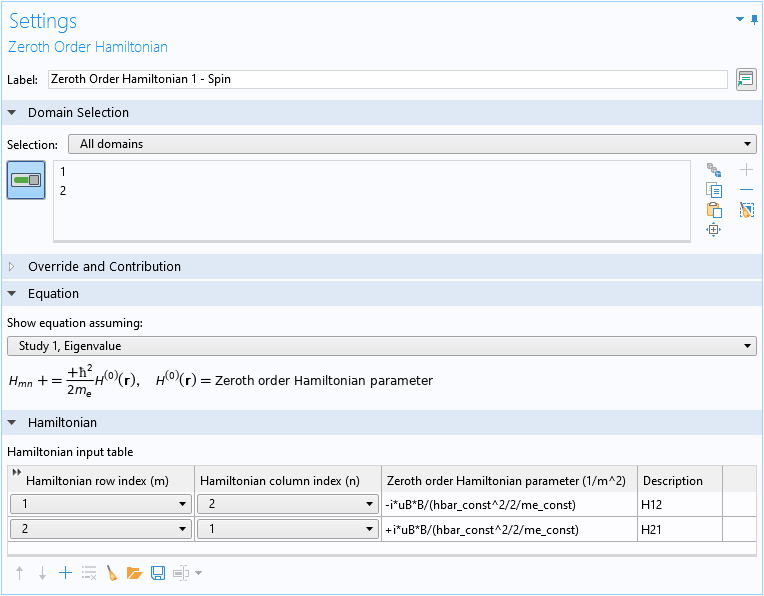(4)

\mu_B \mathbf{B} \cdot \mathbf{\sigma}
= \mu_B B \, \mathbf{n}_y \cdot \mathbf{\sigma}
= \mu_B B \sigma_y
= \left( \begin{array}{cc}
0 & -i\, \mu_B B \\
+i\, \mu_B B & 0 \end{array} \right)

### 理解结果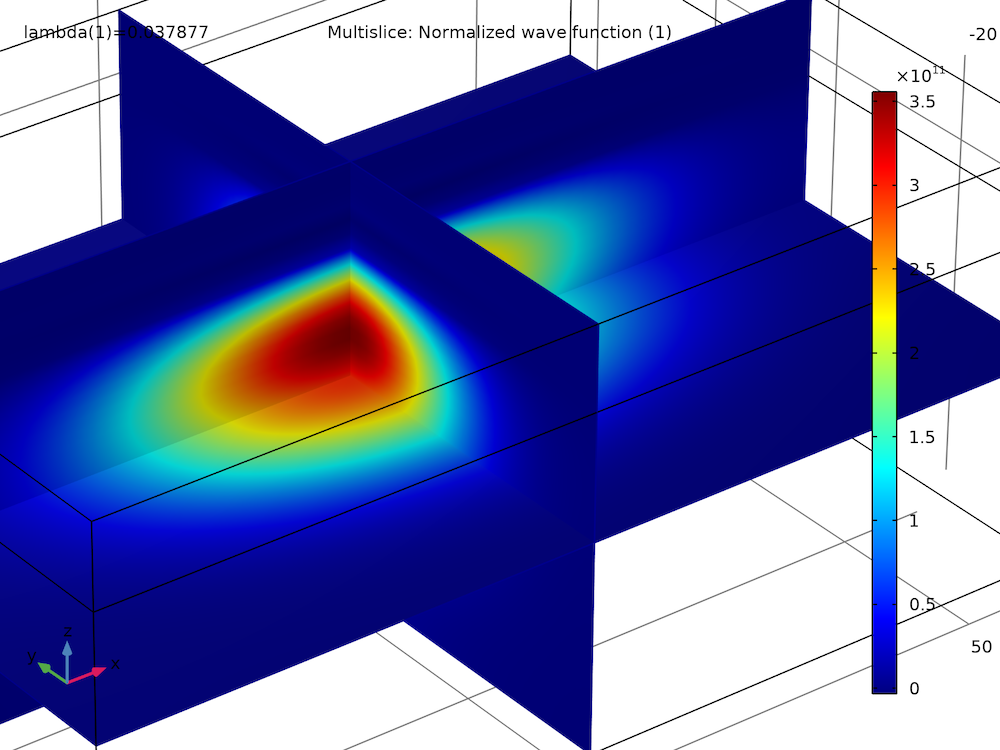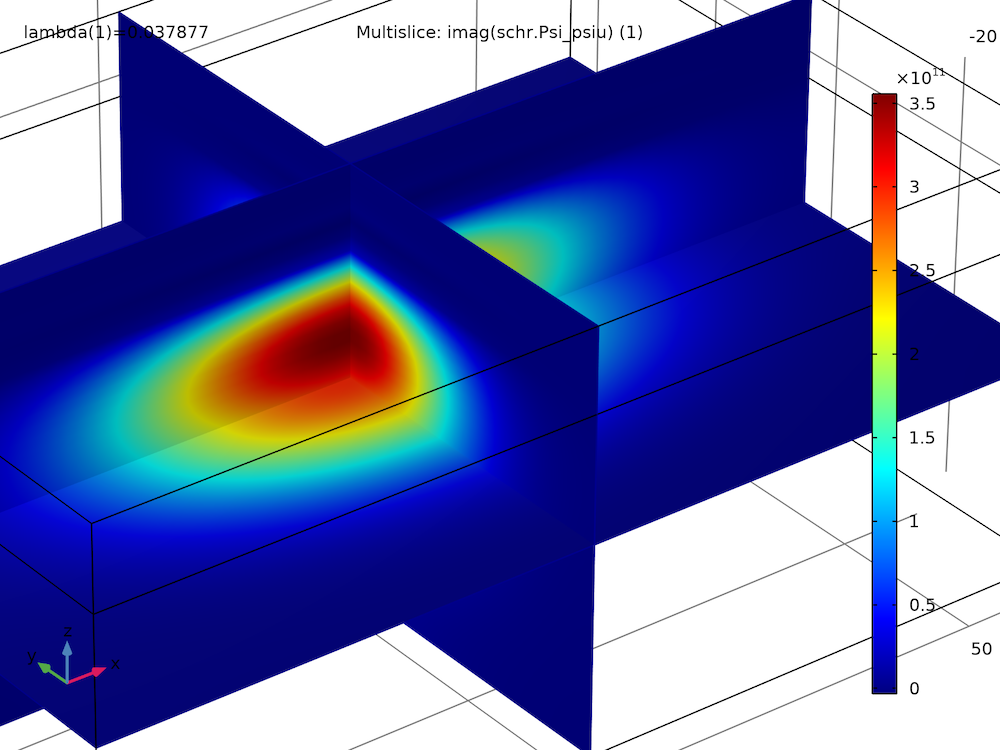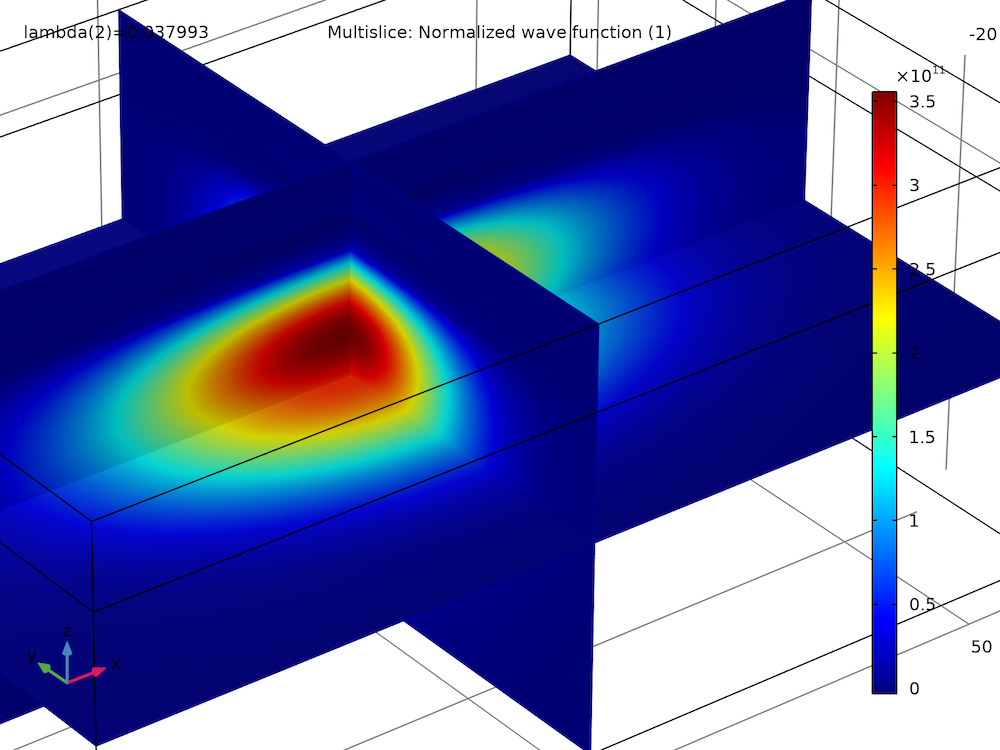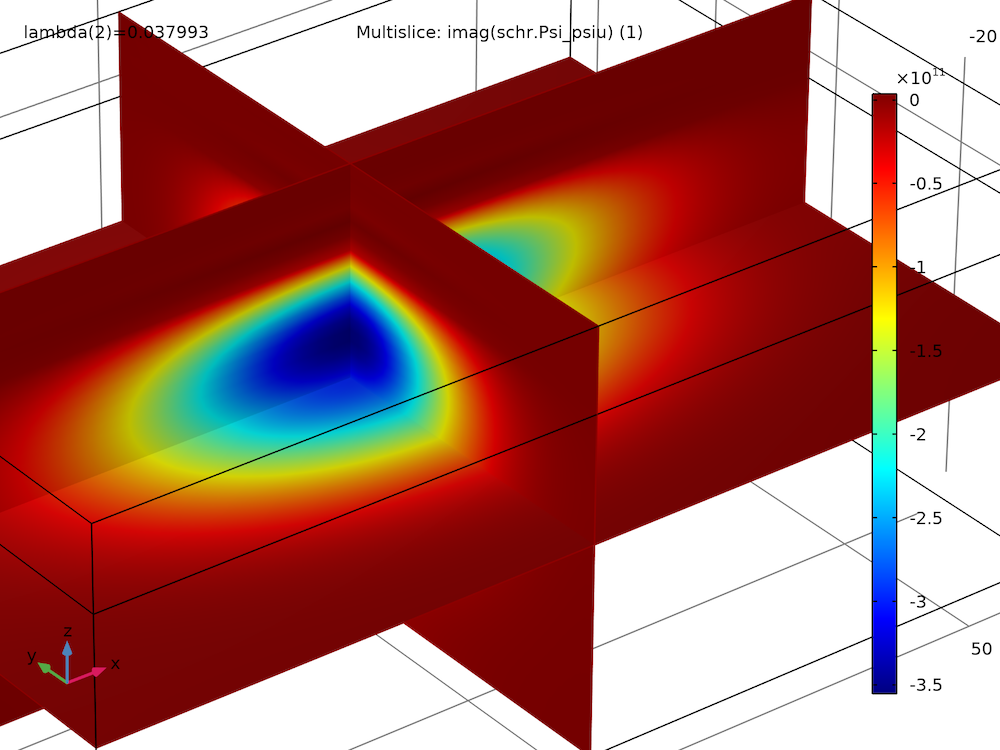特征值 向上（1），点：（0，0，-2） 向下（1），点：（0，0，-2） 0.03788 1.000 + 1.000i 1.000-1.000i 0.03799 1.000-1.000i 1.000 + 1.000i

0.03788 0.1158 0.1158

### 概率密度和动量密度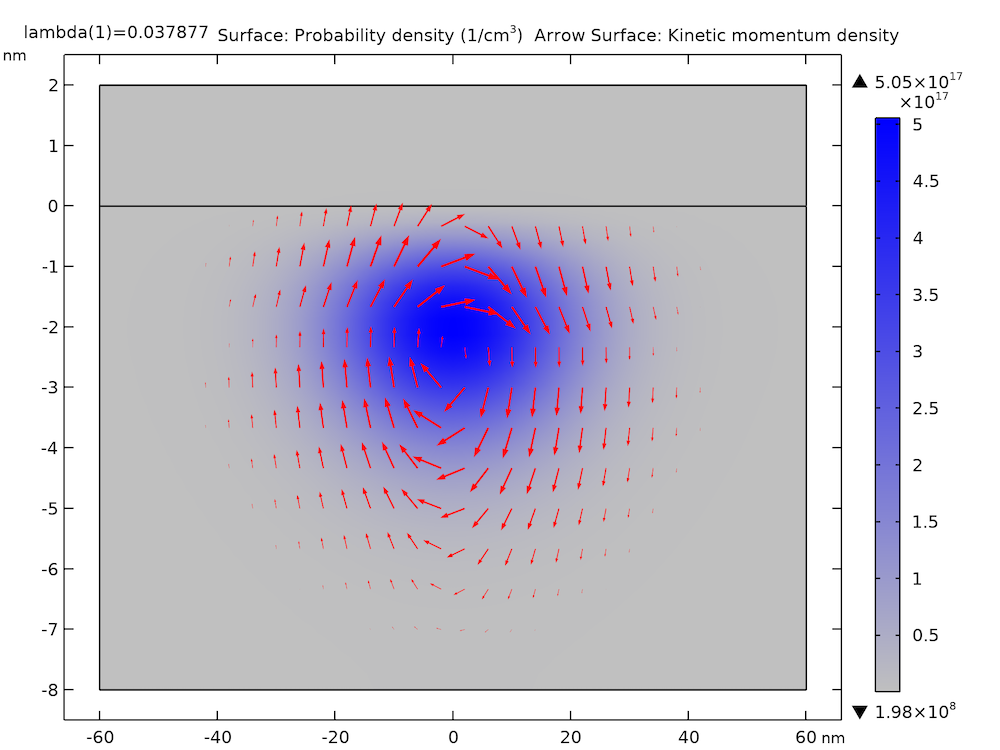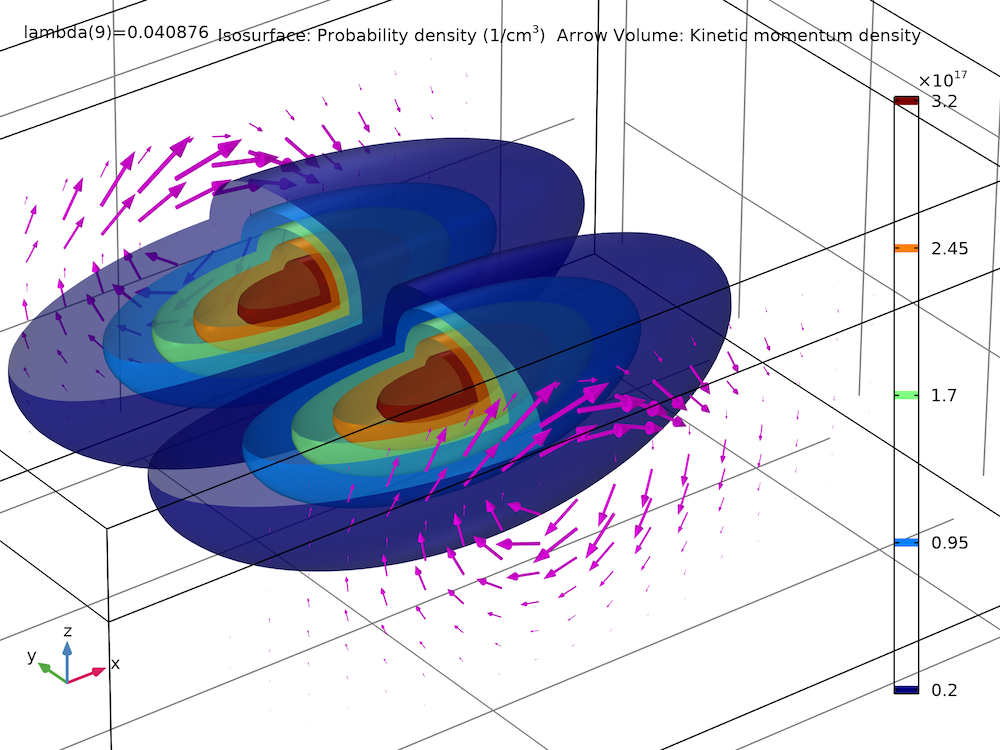### 动手尝试

1. R.M. Jock et al., “A silicon metal-oxide-semiconductor electron spin-orbit qubit,” Nature Communications, 9:1768, 2018.